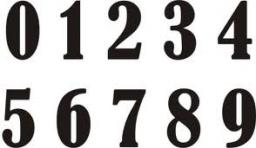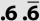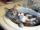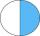# Valid number

Round the 453874528 on 2 significant numbers.

x =  450000000

### Step-by-step explanation:

$x=453874528\doteq 450000000$Did you find an error or inaccuracy? Feel free to write us. Thank you!Tips to related online calculators
Do you want to round the number?

## Related math problems and questions:

• Rounding 2Round the following negative number: -143.021
• Round 9Round number 0.2375 TO 2 SIGNIFICANT FIGURES
• RoundingDouble round number 727, first to tens, then to hundreds. (double rounding)
• A number 2A number decreased by the difference between four and the number.Add this two mixed numbers: 1 5/6 + 2 2/11=
• Expression 1What is 7+8-(5×2)+5-4+(6×(5-3)+6)-(8+10)-7+6?
• Expression 6Evaluate expression: -6-2(4-8)-9
• Evaluate expressionIf x=2, y=-5 and z=3 what is the value of x-2y
• FractionsSort fractions z1 = (6)/(11); z2 = (10)/(21); z3 = (19)/(22) by its size. Result write as three serial numbers 1,2,3.Add two mixed fractions: 2 4/6 + 1 3/6
• NumberDetermine unknown number if you know that difference between five times and triple of number is 42.
• Expression plus minusEvaluate expression: (-1)2 . 12 – 6 : 3 + (-3) . (-2) + 22 – (-3) . 2
• The smallest numberWhat is the smallest number that can be divided by both 5 and 7
• CatsTwo cats caught two mice in two days. How many mouses will catch 6 cats for 6 days?Add and write the result again as hours, minutes, seconds: 2hodiny45min15s + 1h20m50s =Calculate the missing denominator x: (264)/(96) = (11)/(x)If 3t-7=5t, then 6t=